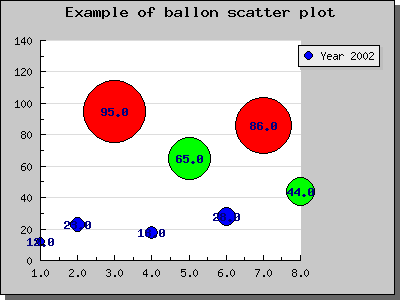Contents Previous Next

## 8.17 Using callbacks for Plot marks

An interesting enhancement when using Plotmarks is the possibility to add a callback function to control the size and color of the plotmarks.

This callback function will get called with the current Y-value (for the plotmark) as it's argument. As return value the callback function must return an array containing three (possible null) values. The values returned must be

1. Plot mark Weight
2. Plot mark Color
3. Plot mark Fill color

The exact meaning of the parameters will of course depend on the type of plot marks being used.

The callback must be a global function and is installed with a call to PlotMark::SetCallback()

So for example to install a callback that changes the fill color for all marks with a (Y) value higher than 90 you could add the lines

90) \$fcolor="red" else \$fcolor=""; return array("","",\$fcolor); } ... \$plot->mark->SetCallback("MarkCallback"); ...'; ShowCodeSnippet(\$t); ?>

As you can see in the above example we have left some of the return values blank. Doing this will just ignore any change of these value and use the global settings for the plotmarks.

If you also let the (Y) value affect the size of the plot marks you can get what is sometimes known as a "balloon plot". The example below is basically a scatter plot that uses filled circles to mark the points. A format callback is then used to change the color and size depending on the Y-value for each plot.Figure 92: Creating a balloon plot by using plot mark callback function [src]

Contents Previous Next